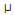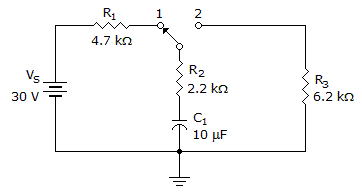# Electronics - Capacitors

### Exercise :: Capacitors - General Questions

31.

If C1, a 4.7F capacitor, and C2, a 3.3F capacitor are in series with 18 Vdc applied, what is the voltage across C1?

 A. 3.3 V B. 7.4 V C. 6.6 V D. 9.4 V

Explanation:

No answer description available for this question. Let us discuss.

32.

What is the impedance of a series RC circuit that consists of a 0.22F capacitor and a 200-ohm resistor connected to a 3 kHz source?

 A. 214 ohms B. 313 ohms C. 414 ohms D. 880 ohms

Explanation:

No answer description available for this question. Let us discuss.

33.

What is the reactance value for a capacitor installed in a dc circuit?

 A. zero B. moderate C. infinite D. cannot tell

Explanation:

No answer description available for this question. Let us discuss.

34.

In a purely capacitive circuit,

 A. current leads voltage by 90° B. voltage leads current by 90° C. current lags voltage by 90° D. current and voltage have a phase relationship of 0°

Explanation:

No answer description available for this question. Let us discuss.

35.How long would it take the capacitor in the given circuit to completely discharge if the switch was in position 2?

 A. 22 ms B. 69 ms C. 345 ms D. 420 ms